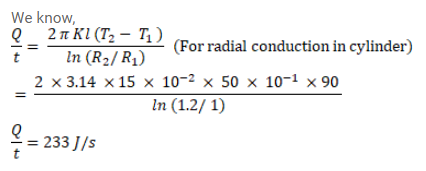# Steam at 120°C is continuously passed through a 50cmQuestion:

Steam at $120^{\circ} \mathrm{C}$ is continuously passed through a $50 \mathrm{~cm}$ long rubber tube of inner and outer radii $1.0 \mathrm{~cm}$ and $1.2 \mathrm{~cm}$. The room temperature is $30^{\circ} \mathrm{C}$. Calculate the rate of heat flow through the walls of the tube. Thermal conductivity of rubber $=0.15 \mathrm{~J} / \mathrm{m}-\mathrm{s}^{\circ} \mathrm{C}$.

Solution: